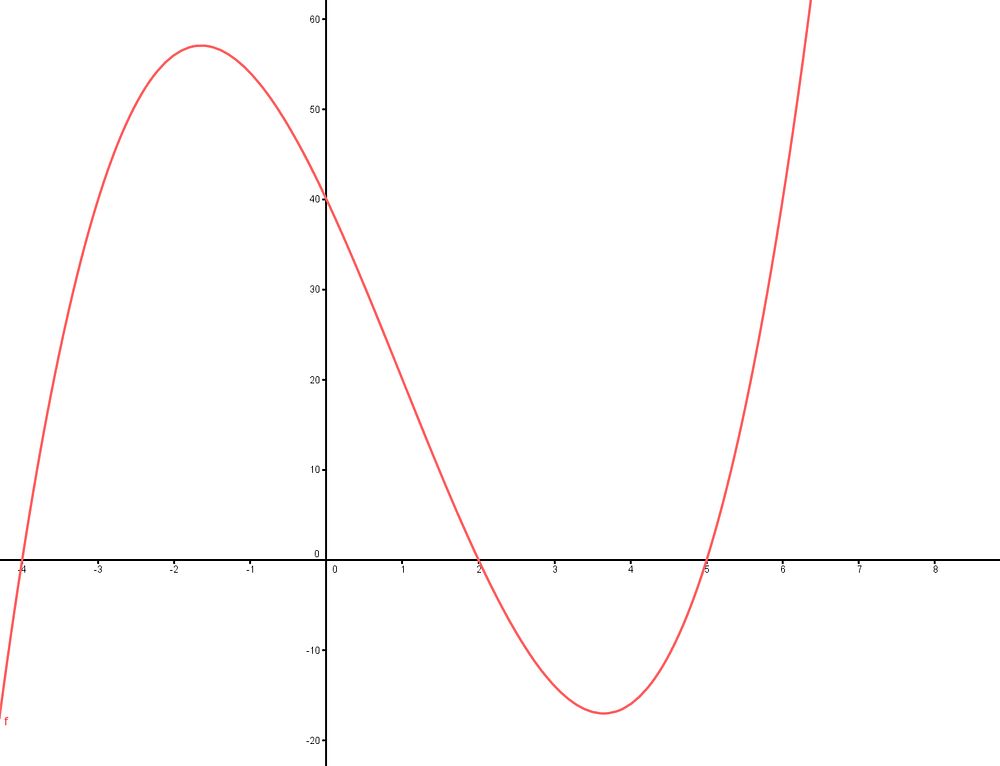# POLYNOMIAL REGRESSION

Source: Deep Learning on MediumLINEAR VS NON-LINEAR REGRESSION

INTRODUCTION :

In this blog we will see how to fit a perfect plane for a dataset . Sometimes it’s sufficient to fit Linear plane . But for sometimes it’s not sufficient to get the good accuracy score by fitting a linear plane . At that condition we go for a Non-Linear plane that best fits our dataset .

We will see which best fits either linear or non-linear plane for our simple dataset for better understanding ?